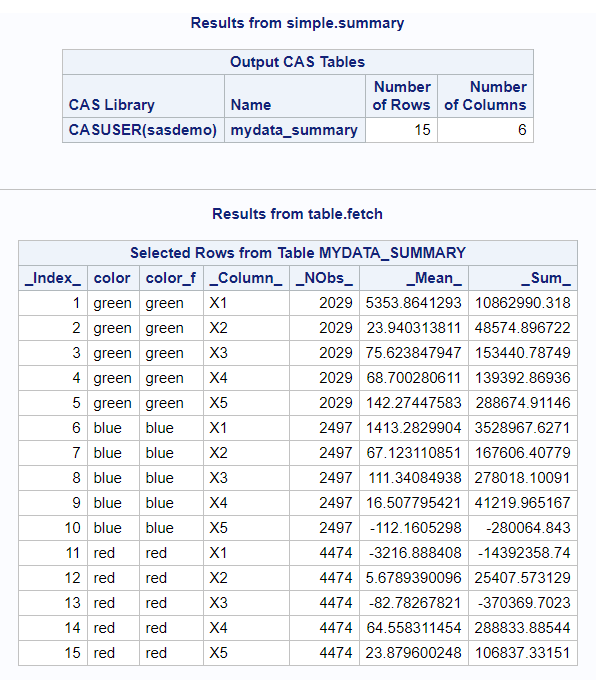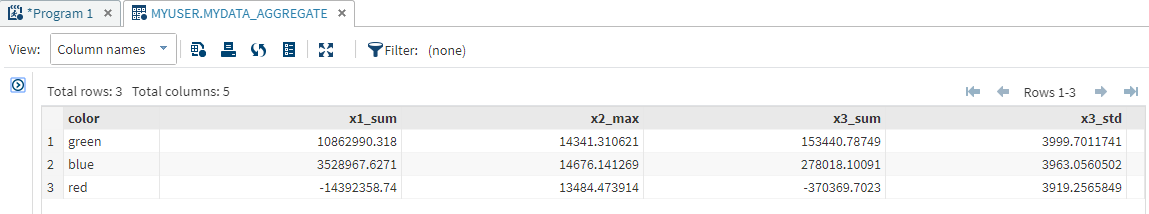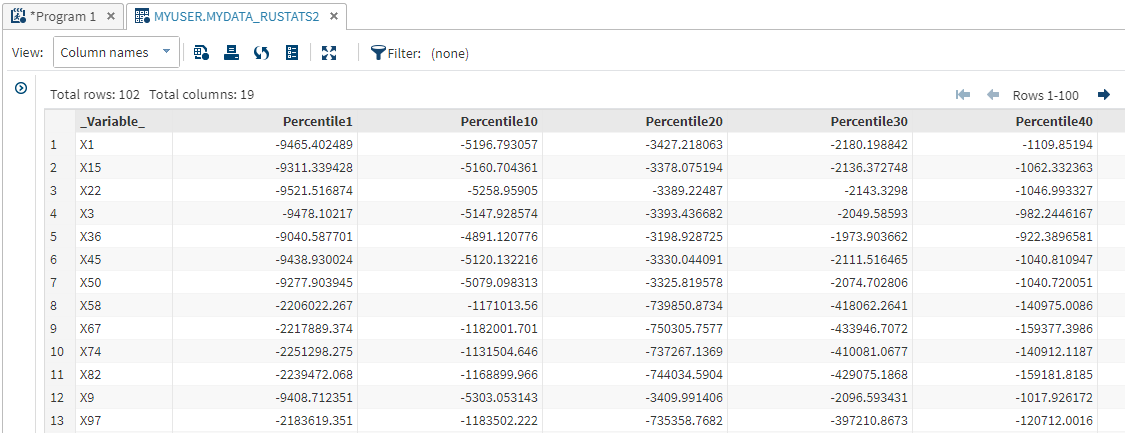Some key components of CASL are the action statements. These statements perform tasks that range from configuring the connection to the server, to summarizing large amounts of data, to processing image files. Each action has its own purpose. However, there is some overlapping functionality between actions. For example, more than one action can summarize numeric variables.

This blog looks at three actions: SIMPLE.SUMMARY, AGGREGATION.AGGREGATE, and DATAPREPROCESS.RUSTATS. Each of these actions generates summary statistics. Though there might be more actions that generate the same statistics, these three are a good place to start as you learn CASL.

### Create a CAS table for these examples

The following step generates a table called mydata, stored in the casuser caslib, that will be used for the examples in this blog.

```cas; libname myuser cas caslib='casuser'; data myuser.mydata; length color \$8; array X{100}; do k=1 to 9000; do i=1 to 50; X{i} = rand('Normal',0, 4000); end; do i=51 to 100; X{i} = rand('Normal', 100000, 1000000); end; if x1 < 0 then color='red'; else if x1 < 3000 then color='blue'; else color='green'; output; end; run;```

### SIMPLE.SUMMARY

The purpose of the Simple Analytics action set is to perform basic analytical functions. One of the actions in the action set is the SUMMARY action, used for generating descriptive statistics like the minimum, maximum, mean, and sum.

This example demonstrates obtaining the sum, mean, and n statistics for five variables (x1–x5) and grouping the results by color. The numeric input variables are specified in the INPUTS parameter. The desired statistics are specified in the SUBSET parameter.

```proc cas; simple.summary / inputs={"x1","x2","x3","x4","x5"}, subset={"sum","mean","n"}, table={caslib="casuser",name="mydata",groupBy={"color"}}, casout={caslib="casuser", name="mydata_summary", replace=true}; run; table.fetch / table={caslib="casuser",name="mydata_summary" }; run; quit;```

The SUMMARY action creates a table that is named mydata_summary. The TABLE.FETCH action is included to show the contents of the table.The mydata_summary table can be used as input for other actions, its variable names can be changed, or it can be transposed. Now that you have the summary statistics, you can use them however you need to.

### AGGREGATION.AGGREGATE

Many SAS® procedures have been CAS-enabled, which means you can use a CAS table as input. However, specifying a CAS table does not mean all of the processing takes place on the CAS server. Not every statement, option, or statistic is supported on the CAS server for every procedure. You need to be aware of what is not supported so that you do not run into issues if you choose to use a CAS-enabled procedure. In the documentation, refer to the CAS processing section to find the relevant details.

When a procedure is CAS-enabled, it means that, behind the scenes, it is submitting an action. The MEANS and SUMMARY procedure steps submit the AGGREGATION.AGGREGATE action.

With PROC MEANS it is common to use a BY or CLASS statement and ask for multiple statistics for each analysis variable, even different statistics for different variables. Here is an example:

```proc means sum data=myuser.mydata noprint;
by color;
var x1 x2 x3;
output out=test(drop=_type_ _freq_) sum(x1 x3)=x1_sum x3_sum
max(x2)=x2_max std(x3)=x3_std;
run;
```

The AGGREGATE action produces the same statistics and the same structured output table as PROC MEANS.

```proc cas; aggregation.aggregate / table={name="mydata",caslib="casuser",groupby={"color"}} casout={name="mydata_aggregate", caslib='casuser', replace=true} varspecs={{name='x1', summarysubset='sum', columnnames={'x1_sum'}}, {name='x2', agg='max', columnnames={'x2_max'}}, {name='x3', summarysubset={'sum','std'}, columnnames={'x3_sum','x3_std'}}} savegroupbyraw=true, savegroupbyformat=false, raw=true; run; quit;```

The VARSPECS parameter might be confusing. It is where you specify the variables that you want to generate statistics for, which statistics to generate, and what the resulting column should be called. Check the documentation: depending on the desired statistic, you need to use either SUMMARYSUBSET or AGG arguments.

If you are using the GROUPBY action, you most likely want to use the SAVEGROUPBYRAW=TRUE parameter. Otherwise, you must list every GROUPBY variable in the VARSPECS parameter. Also, the SAVEGROUPBYFORMAT=FALSE parameter prevents the output from containing _f versions (formatted versions) of all of the GROUPBY variables.### DATAPREPROCESS.RUSTATS

The RUSTATS action, in the Data Preprocess action set, computes univariate statistics, centralized moments, quantiles, and frequency distribution statistics. This action is extremely useful when you need to calculate percentiles. If you ask for percentiles from a procedure, all of the data will be moved to the compute server and processed there, not on the CAS server.

This example has an extra step. Actions require a list of variables, which can be cumbersome when you want to generate summary statistics for more than a handful of variables. Macro variables are a handy way to insert a list of strings, variable names in this case, without having to enter all of the names yourself. The SQL procedure step generates a macro variable containing the names of all of the numeric variables. The macro variable is referenced in the INPUTS parameter.

The RUSTATS action has TABLE and INPUTS parameters like the previous actions. The REQUESTPACKAGES parameter is the parameter that allows for a request for percentiles.

The example also contains a bonus action, TRANSPOSE.TRANSPOSE. The goal is to have a final table, mydata_rustats2, with a structure like PROC MEANS would generate. The tricky part is the COMPUTEDVARSPROGRAM parameter.

The table generated by the RUSTATS action has a column called _Statistic_ that contains the name of the statistic. However, it contains “Percentile” multiple times. A different variable, _Arg1_, contains the value of the percentiles (1, 10, 20, and so on). The values of _Statistic_ and _Arg1_ need to be combined, and that new combined value generates the new variable names in the final table.

The COMPUTEDVARS parameter specifies that the name of the new variable will hold the concatenation of _Statistic_ and _Arg1_. The COMPUTEDVARSPROGRAM parameter tells CAS how to create the values for NEWID. The NEWID value is then used in the ID parameter to make the new variable names—pretty cool!

```proc sql noprint; select quote(strip(name)) into: numvars separated by ',' from dictionary.columns where libname='MYUSER' and memname='MYDATA' and type='num'; quit;   proc cas; dataPreprocess.rustats / table={name="mydata",caslib="casuser"} inputs={&numvars} requestpackages={{percentiles={1, 10, 20, 30, 40, 50, 60, 70, 80, 90, 95 99},scales={"std"}}} casoutstats={name="mydata_rustats",caslib="casuser"} ;   transpose.transpose / table={caslib='casuser', name="mydata_rustats", groupby={"_variable_"}, computedvars={{name="newid",format="\$20."}},computedvarsprogram="newid=strip(_statistic_)||compress(strip(_arg1_),'.-');"} transpose={"_value_"} id={"newid"} casOut={caslib='casuser', name="mydata_rustats2", replace=true}; run; quit;```

Here is a small portion of the final table. Remember, you can use the TABLE.FETCH action to view the table contents as well.### Summary

Summarizing numeric data is an important step in analyzing your data. CASL provides multiple actions that generate summary statistics. This blog provided a quick overview of three of those actions: SIMPLE.SUMMARY, AGGREGATION.AGGREGATE, and DATAPREPROCESS.RUSTATS.

The wonderful part of so many choices is that you can decide which one best fits your needs. Summarizing your data with actions also ensures that all of the processing occurs on the CAS server and that you are taking full advantage of its capabilities.

Be sure to use the DROPTABLE action to delete any tables that you do not want taking up space in memory:

```proc cas; table.droptable / caslib='casuser' name='mydata' quiet=true; table.droptable / caslib='casuser' name='mydata_summary' quiet=true; table.droptable / caslib='casuser' name='mydata_aggregate' quiet=true; table.droptable / caslib='casuser' name='mydata_rustats' quiet=true; table.droptable / caslib='casuser' name='mydata_rustats2' quiet=true; quit; cas casauto terminate;```